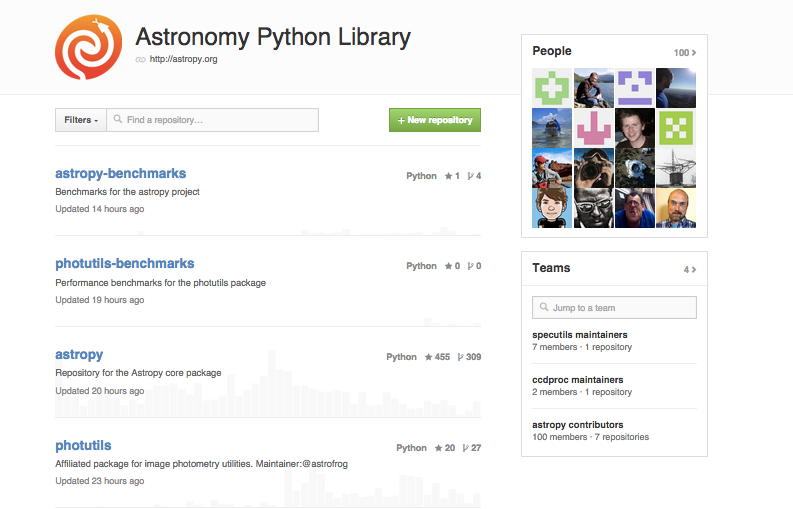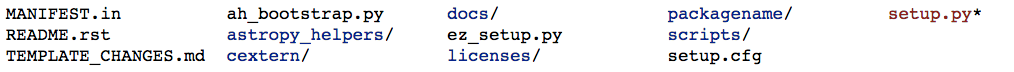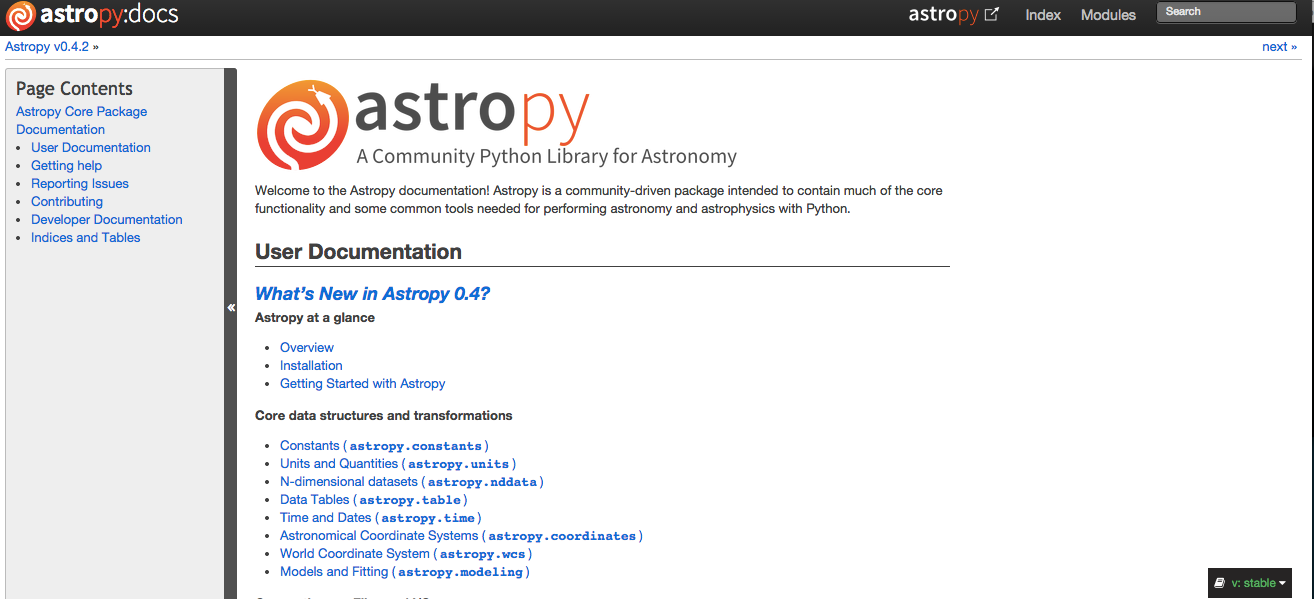## Steve Crawford

(one small member of the much larger astropy community)

# Contributors to Astropy

## Project Coordinators

### Astropy on Github# Astropy Core

## v0.4.2

Only has Numpy as a required dependency (easy to install)

Well tested, documented, and stable code

Works on Linux, MacOS X, and Windows

Python 2 and 3 compatible

Latest stable release v0.4.2 on Sept 23

### Core data structures and transformations

• Constants
• Units and Quantities
• N-dimensional datasets
• Data Tables
• Time and Dates
• Astronomical Coordinate Systems
• World Coordinate System
• Models and Fitting

• ### Connecting up: Files and I/O

• FITS File handling
• ASCII Tables
• VOTable XML handling
• Miscellaneous Input/Output

• ### Astronomy computations and utilities

• Convolution and filtering
• Cosmological Calculations
• Astrostatistics Tools
• Virtual Observatory Access

• ## Analysis with Units

In :
from astropy import constants as c
c.G

Out:
$6.67384\times 10^{-11} \; \mathrm{\frac{m^{3}}{kg\,s^{2}}}$
In :
M = c.M_earth
M

Out:
$5.9742\times 10^{+24} \; \mathrm{kg}$
In :
from astropy import units as u
r = c.R_earth
v_esc = (2 * c.G * M / r)**0.5
v_esc.to(u.km/u.s)

Out:
$11.1814 \; \mathrm{\frac{km}{s}}$
In :
v_esc = ((2 * c.G * M / (r+559*u.km))**0.5)
v_esc.to(u.km/u.s)

Out:
$10.7214 \; \mathrm{\frac{km}{s}}$
In :
v_esc = ((2 * c.G * 3 * u.m / (r+559*u.km))**0.5)
v_esc.to(u.km/u.s)

---------------------------------------------------------------------------
UnitsError                                Traceback (most recent call last)
<ipython-input-9-871ca449b6fa> in <module>()
1 v_esc = ((2 * c.G * 3 * u.m / (r+559*u.km))**0.5)
----> 2 v_esc.to(u.km/u.s)

/Users/crawford/programs/astropy/astropy/units/quantity.pyc in to(self, unit, equivalencies)
586         unit = Unit(unit)
587         new_val = np.asarray(
--> 588             self.unit.to(unit, self.value, equivalencies=equivalencies))
589         return self._new_view(new_val, unit)
590

/Users/crawford/programs/astropy/astropy/units/core.pyc in to(self, other, value, equivalencies)
924             If units are inconsistent
925         """
--> 926         return self.get_converter(other, equivalencies=equivalencies)(value)
927
928     def in_units(self, other, value=1.0, equivalencies=[]):

/Users/crawford/programs/astropy/astropy/units/core.pyc in get_converter(self, other, equivalencies)
860         except UnitsError:
861             return self._apply_equivalences(
--> 862                 self, other, self._normalize_equivalencies(equivalencies))
863         return lambda val: scale * _condition_arg(val)
864

/Users/crawford/programs/astropy/astropy/units/core.pyc in _apply_equivalences(self, unit, other, equivalencies)
823         raise UnitsError(
824             "{0} and {1} are not convertible".format(
--> 825                 unit_str, other_str))
826
827     def get_converter(self, other, equivalencies=[]):

UnitsError: 'm(3/2) / (kg(1/2) s)' and 'km / s' (speed) are not convertible

## Using Coordinates

In []:
from astropy import units as u
from astropy.coordinates import SkyCoord
c1 = SkyCoord('00h42m00s', '+41d12m00s', 'icrs')

In []:
print("{0} {1}".format( c1.ra,c1.dec))

In []:
print c1.to_string('hmsdms')

In []:
c2 = SkyCoord(ra=11*u.degree, dec=10*u.degree, distance=11.5*u.pc, frame='icrs')
c1.separation(c2)


Also available are coordinate transformation between different systems (e.g. ICRS, FK5, galactic) and matching catalags of coordinates.

## Modeling

Astropy also has a modelling fitting package. Let's see how it works. Let's create a Gaussian shape and add some noise to it.

In []:
import numpy as np
x = np.linspace(-5., 5., 200)
y = 3 * np.exp(-0.5 * (x - 1.3)**2 / 0.8**2)
y += np.random.normal(0., 0.2, x.shape)


Now let's fit the model using the astropy modeling class

In []:
from astropy.modeling import models, fitting
g_init = models.Gaussian1D(amplitude=1., mean=0, stddev=1.)
fit_g = fitting.LevMarLSQFitter()
g = fit_g(g_init, x, y)
print(g)

In []:
import pylab as plt
plt.figure(figsize=(8,5))
plt.plot(x, y, 'ko')
plt.plot(x, g(x), 'r-', lw=2, label='Gaussian')
plt.xlabel('Position')
plt.ylabel('Flux')
plt.legend(loc=2)
plt.show()


A range of different models already exist for 1-D and 2-D fitting. The API for the modeling class is still being refined but it should be stable in the next major release.

In addition to what has been shown so far, a number of core data structures and transformations have been included here to help build and coordinate other packages. These include:
• Quantity: An object with a value and unit
• Table: functionality for storing and manipulating heterogeneous tables of data
• NDData: A general astronomical data object including data, meta, uncertainty, wcs
• Time: functionality for manipulating astronomical relevant times and dates
• WCS: World Coordinate Systems

## Accessing Data

• Tools for accessing the virtual observatory

• In []:
from astropy.vo.client import conesearch

• Tools for reading/writing FITS files (formerly pyfits)

• In []:
from astropy.io import fits

• Tools for reading in ascii tables including CDS, IPAC, HTML, Latex, Sextractor

• In []:
from astropy.io import ascii

In []:
print table


## Cosmology in Astropy

In []:
from astropy.cosmology import WMAP9 as cosmo
cosmo.H(0)

In []:
cosmo


A number of cosmological models are already available and the user can define their own cosmological models including dark energy and neutrino properties

In []:
from astropy.cosmology import WMAP7
from astropy.cosmology import WMAP9 as cosmo
from astropy.cosmology import Planck13

z = np.linspace(0,10,200)

plt.figure(figsize=(8,5))
plt.plot(z, WMAP7.luminosity_distance(z)-cosmo.luminosity_distance(z), 'b-', label='WMAP7')
plt.plot(z, cosmo.luminosity_distance(z)-cosmo.luminosity_distance(z), '-', color='black', label='WMAP9')
plt.plot(z, Planck13.luminosity_distance(z)-cosmo.luminosity_distance(z), 'g-', label='Planck13')
plt.xlabel('z')
plt.ylabel('$\Delta D_L [%s]$' % cosmo.luminosity_distance(0).unit)
plt.legend(loc=2)
plt.show()


## Useful statistics for Astronomy

In []:
from astropy import stats
for x in stats.funcs.__all__: print x


# Astropy Framework

Development of testing, documentation, and installation infrastructre to make it easier to build and deploy your own code

## Package Template# Astropy Affiliated Packages

## Astropy Affiliated Packages

These packages are at different stages of development and there are a number of other packages in early development which are not mentioned here. Some of the packages will be integrated into the core astropy package.

### APLPY

In []:
import aplpy
gc = aplpy.FITSFigure('2MASS_k.fits')
gc.show_rgb('2MASS_arcsinh_color.png')


### astroquery

In []:
from astroquery.simbad import Simbad
result_table.pprint(show_unit=True)


Services available to query include Vizier, Simbad, NED, SDSS, GAMA, archives, catalogs, and many more online resources

### ccdproc

In []:
import ccdproc

In []:
ccd = ccdproc.subtract_overscan(ccd, fits_section=ccd.header['biassec'],
median=True, model=models.Polynomial1D(5))
ccd.write('out.fits', clobber=True)

In []:
fc = aplpy.FITSFigure(ccd.data)
fc.show_grayscale()


# How to get involved

Join astropy@scipy.org

Provide feedback (submit issues, ask questions)

Use the astropy template and astropy classes for your packages

Look for 'easy' issues and submit a pull request

Contribute code either to astropy or an affiliated package

Contacts